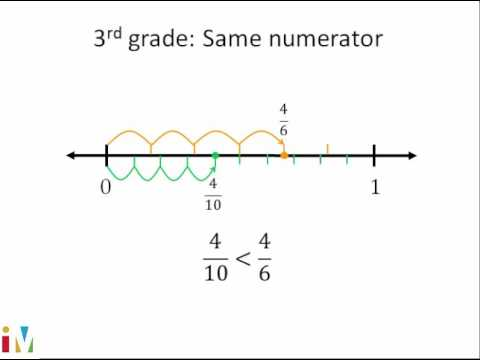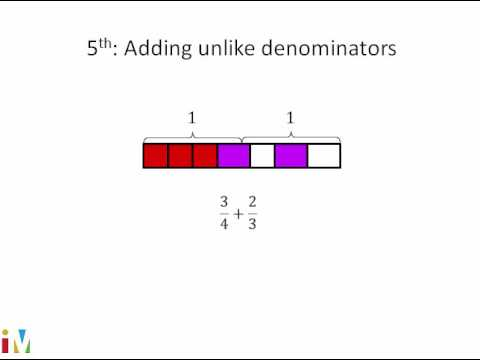Video

# Adding and subtracting 3 fractions (Full video)

Description: Sal solves 1/4 + 3/5 - 3/10 by rewriting each fraction to have a common denominator. So we want to figure out what 1/4 plus 3/5 minus 3/10 is. So the first thing that might jump out at you is look, I have these fractions that I'm adding and subtracting, but they all have different denominators. So in order to add and subtract them in a reasonable way, we'd want to rewrite them so they all have the same denominator.

### Other videos you might be interested in### Comparing Fractions

#### Illustrative Mathematics